# HBSE 10th Class Science Solutions Chapter 11 Human Eye and Colourful World

Haryana State Board HBSE 10th Class Science Solutions Chapter 11 Human Eye and Colourful World Textbook Exercise Questions and Answers.

## Haryana Board 10th Class Science Solutions Chapter 11 Human Eye and Colourful World

HBSE 10th Class Science Human Eye and Colourful World Textbook Questions and Answers

Question 1.
The human eye can focus on objects at different distances by adjusting the focal length of the eye lens. This is due to
(a) Presbyopia
(b) Accommodation
(c) Near-sightedness
(d) Far-sightedness
(b) AccommodationQuestion 2.
The human eye forms the image of an object at its ……….
(a) cornea
(b) iris
(c) pupil
(d) retina
(d) retina

Question 3.
The least distance of distinct vision for a young adult with normal vision is about
(a) 25 m
(b) 2.5 cm
(c) 25 cm
(d) 2.5 m
(c) 25 cm

Question 4.
The change in focal length of an eye lens ¡s caused by the action of the
(a) pupil
(b) retina
(c) cillary muscles
(d) Iris
(c) cillary musclesQuestion 5.
A person needs a lens of power – 5.5 diopters for correcting his distant vision. For correcting his near vision he needs a lens of power +1.5 diopter. What is the focal length of the lens required for correcting (i) distant vision, and (ii) near vision?
1. Power of distance viewing part of the lens, Pi = – 5.5 D
Focal length of this part, f1 = $$\frac{1}{P_1}=\frac{1}{-5.5}$$ = m = – 18.73 cm (concave lens)

2. The power of the near-vision part is measured relative to the main part of the lens of power – 5.5
∴ P1+P2 = P or – 5.5+P2= + 1.5 or P2=+6.5 D
Focal length of near-vision part, f2 = $$\frac{1}{P_2}=\frac{1}{+6.5}$$ m = m = + 15.4 cm (convex lens)

Question 6.
The far point of a myopic person is 80 cm in front of the eye. What is the nature and power of the lens required to correct the problem?
The lens should be able to make the objects at infinity appear at the far point.
∴ For object at infinity, µ = – ∞
Far point distance of the defected eye, v = – 80 cm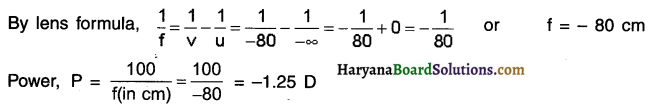A negative sign shows that the lens is a concave lens.
Thus a concave lens of power – 1.25 D is needed to correct the problem.Question 7.
Make a diagram to show how hypermetropia is corrected. The near point of a hypermetropic eye is 1 m. What is the power of the lens required to correct this defect? Assume that the near point of the normal eye is 25 cm.
The object placed at 25 cm from the correcting lens must produce a virtual image at 1 m or 100 cm.
u = – 25 cm, y = -100 cm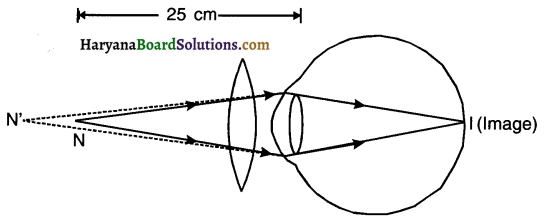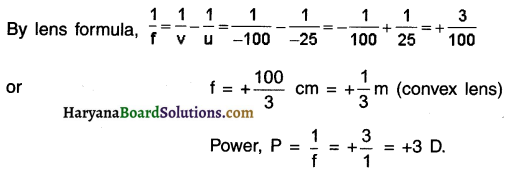Question 8.
Why is a normal eye not able to see clearly the objects placed closer than 25 cm?
At distance less than 25 cm, the ciliary muscles are unable to bulge the eye lens any more. So, the objects cannot be focused on the retina and thus appears blurred.

Question 9.
What happens to the Image distance in the eye when we increase the distance of an object from the eye?
1. When we increase the distance of an object from the eye there does not occur any change in the image distance.
2. When the object is moved farther, the ciliary muscles get relaxed and the eye lens become thin. This increases the focal length and the image looks clear.Question 10.
Why do stars twinkle?
1. Density of atmosphere is not uniform everywhere.
2. There are different layers of atmosphere with different refractive indices.
3. Atmospheric layer at lower altitude is colder P and denser compared to layer at higher altitude.
4. When the light travels from star at a rarer medium towards earth at denser medium, it bends towards normal.
5. Thus, due to refraction towards normal, the position of star appears higher from its actual position.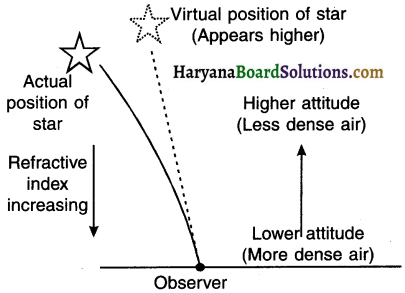Reason for twinkling of stars:

• The physical condition of refracting medium e. the earth’s atmosphere is not stationary. Hence, the position of star seems to be changing continuously.
• This results in the continuous change in the path of the rays coming from the stars and continuous change in the intensity of light. This leads to twinkling of stars.
• Light from a star is refracted or say bent as it leaves the space and enters the earth’s atmosphere.
• Air at higher altitude is rare whereas near the earth’s surface it is dense.
• As a result, when light from the star comes down, the dense air bends the light more.
• Due to this, refraction of star’s light, the star appears to be at a higher position than it actually is.Question 11.
Explain why the planets do not twinkle.
1. The stars are very far from us and so they may be considered as the point sources of light.
2. Compared to stars, planets are much nearer to the earth and so planets appear quite big.
3. Hence, planets cannot be considered a single point source of light but a collection of a very large number of point sources of light.
4. Thus, on the whole, the brightness of the planet always remains the same and it does not appear twinkle. But the atmospheric refraction affects stars more since they are point sources of light and so stars twinkle.

Question 12.
Why does the Sun appear reddish early in the morning?
1. White light coming from the sun has to travel a large distance in the atmosphere before reaching to the observer.
2. During sunrise or sunset, most of the blue colour present in sunlight has been scattered out and it is away from our sight.
3. As a result, only red light remains present in the beam of sunlight and so only red colour reaches our eye.
4. As a result, sun appears reddish at sunrise and sunset.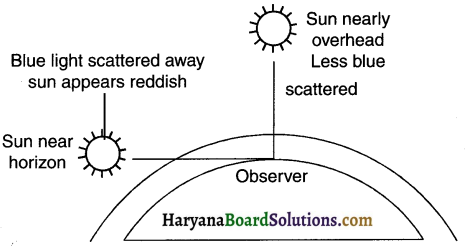Question 13.
Why does the sky appear dark instead of blue to an astronaut?
1. The atmosphere becomes thinner as one moves above the earth.
2. Astronauts travel in the space which is at very high altitudes. At such altitudes, the sun cannot scatter any sunlight because of extremely thin atmosphere. As a result, the sky appears dark and not blue to the astronauts.

HBSE 10th Class Science Human Eye and Colourful World InText Activity Questions and Answers

Textbook Page no – 190

Question 1.
What is meant by power of accommodation of the eye?
The ability of the eye lens to adjust its focal length as per the requirement so that the objects can be seen clearly is called the power of accommodation of eyes.Question 2.
A person with a myopic eye cannot see objects beyond 1.2 m distinctly. What should be the type of the corrective lens used to restore proper vision?
The person should use diverging i.e. concave lens.

Question 3.
What is the far point and near point of the human eye with normal vision?
For a human eye with normal vision, the near point is 25 cm lron the eyes whereas the far point is at infinity.

Question 4.
A student has difficulty reading the blackboard while sitting in the last row. What could be the defect the child is suffering from? How can it be corrected?
1. Since the student is sitting in the last row, the blackboard is at a far distance. The student is unable to read at far distance means he is suffering from myopia i.e. near-sightedness.
2. The student should use a concave length of suitable focal length to cure this problem.

Activities

Activity – 1

1. Fix a sheet of white paper on a drawing board using drawing pins.
2. Place a glass prism on it in such a way that it rests on its triangular base. Trace the outline of the prism using a pencil.
3. Draw a straight line PE inclined to one of the refracting surfaces, say AB, or the prism.
4. Fix two pins, say at points P and Q, on the line PE as shown in Figure.
5. Look for the images of the pins, fixed at P and Q, through the other face AC.
6. Fix two more pins, at points R and S, such that the pins at R and S and the images of the pins at P and Q lie on the same straight line.
7. Remove the pins and the glass prism.
8. The line PE meets the boundary of the prism at point E. Similarly join and produce the points R and S. Let these lines meet the boundary of the prism at E and F, respectively. Join E and F.
9. Draw a perpendicular to the refracting surfaces AB and AC of the prism at point E and F, respectively. Mark the angle of incidence (∠i), the angle of refraction (∠r) and the angle of emergence (∠e) as shown in figure.
10. Discuss the refraction of light through the prism. Try to find a relation between angles i, e, A and D.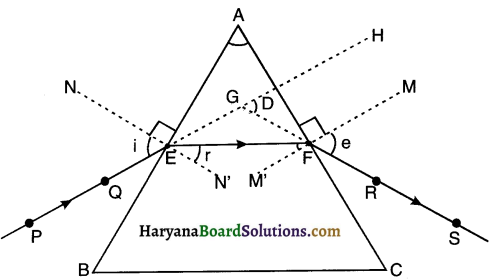Observation and conclusion:

1. The incident ray PE undergoes refraction on hitting the face AB and bends towards the normal NN’ along the path EF. Again as ray EF retracts from glass to air at face AC, it bends away from the normal MM’. Thus, the emergent ray FS bends towards the base of the prism.2. We measure the angle of incidence i, angle of prism A, angle of emergence e and the angle of deviation D. On measuring, we can see that, i+e=A+D

Activity 2.

1. Take a thick sheet of cardboard and make a small hole or narrow slit in its middle.
2. Allow sunlight to fall on the narrow slit. This gives a narrow beam of white light.
3. Now, take a glass prism and allow the light from the slit to fall on one of its faces as shown in Fig.
4. Turn the prism slowly until the light that comes out of it appears on a nearby screen.
5. What do you observe? You will find a beautiful band of colours. Why does this happen?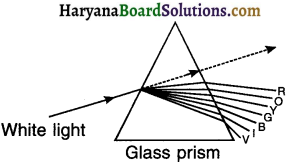Observation and conclusion:

• The glass prism splits the incident white light into a band of seven colours.
• The band of colour called spectrum is made up of Violet, Indigo, Blue, Green, Yellow, Orange and Red colours in the said sequence. This is an example of dispersion of light.
• Red coloured light shows least deviation where as violet-coloured light shows maximum deviation.Activity 3.

1. Place a strong source (S) of white light at the focus of a converging lens (L1). This lens provides a parallel beam of light.
2. Allow the light beam to pass through a transparent glass tank (T) containing clear water.
3. Allow the beam of light to pass through a circular hole (C) made in a cardboard.
Obtain a sharp image of the circular hole on a screen (MN) using a second converging lens (L2), as shown in Figure.
4. Dissolve about 200 g of sodium thiosulphate (hypo) in about 2 L of clean water taken in the tank.
Add about 1 to 2 mL of concentrated sulphuric acid to the water. What do you observe?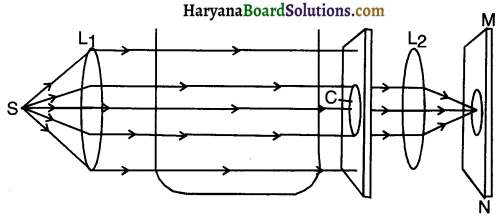Observation and conclusion:

• The fine micro-particles will precipitate in few minutes.
• As the sulphur (colloids) particles begin to form, we will observe that blue light starts appearing from
three sides of the tank. This demonstrates scattering of light.
• On observing the tank from the circular hole side, we can first see an image of orange colour and
then of bright crimson red colour on the screen.
• Thus, the colloidal sulphur particles scatter the blue component of white light to maximum extent
which can be seen from three sides of the tank.
• The least scattered red component gets transmitted through the colloidal solution and reaches the screen.